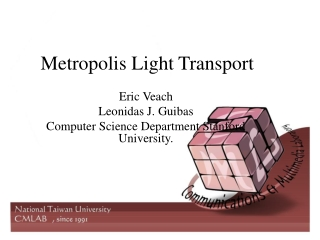DownloadDownload PresentationMetropolis Light Transport

# Metropolis Light Transport

Download Presentation## Metropolis Light Transport

- - - - - - - - - - - - - - - - - - - - - - - - - - - E N D - - - - - - - - - - - - - - - - - - - - - - - - - - -
##### Presentation Transcript

1. Metropolis Light Transport Eric Veach Leonidas J. Guibas Computer Science Department Stanford University.

2. Outline • Prerequisite • Overview • Initial Path • Mutation • Result

3. Markov chain • Markov chain : • A stochastic process with Markov property. The range of random variable X is called the state space. is called the transition probability. (one step)

4. Markov chain How about two or three steps? Distribution over states at time n+1

5. Markov chain • Stationary distribution (steady-state distribution):

6. Markov chain • Ergodic theory: • If we take stationary distribution as initial distribution...the average of function f over samples of Markov chain is :

7. Markov chain • If the state space is finite...transition probability can be represented as a transition matrix P.

8. Overview Rendering equation Measurement equation Expand L by rendering equation

9. Overview μ fj

10. Overview • f : the energy of all image plane. • fj : the energy of pixel j. We can think wj restricted f to the pixel j.

11. Overview Now, we want to solve the integration :

12. Overview • As the Monte-Carlo Method, we want the probability distribution p proportional to f. K is transition function converge The same idea ..in this paper, we want stationary distribution Proportional to f.

13. Overview • If we let And we prefer the stationary distribution propose to f Mutation determine T(y|x). If we determine a mutation, we must Calculate a(y|x) to satisfy this equation!

14. Overview In this paper, based on bidirectional ray tracing Based on three strategies, decide T(y|x) Computed by T(y|x)

15. Initial Path In this paper, based on bidirectional ray tracing Based on three strategies, decide T(y|x) Computed by T(y|x)

16. Initial Path • How to choose Initial Path? (Have a good start!) • Run n copies of the algorithm and accumulate all samples into one imege. • Sample n paths : • Resample form the n paths to obtain relative small number n’ paths. • If n’ = 1, then just take the mean value.

17. Mutation In this paper, based on bidirectional ray tracing Based on three strategies, decide T(y|x) Computed by T(y|x)

18. Properties of good mutations • High acceptance probability. • Prevent the path sequence x, x, x, x, x, x, … • Large changes to the path. • Prevent path sequence with high correration • Ergodicity. • Ensure random walk converge to an ergodic state.

19. Properties of good mutations • Changes to the image location. • Minimize correlation between image plane. • Stratification. • Uniform distribute on image plane. • Low cost. • As the word says..

20. Mutation • In this paper, three Mutation strategies is presented… • Bidirectional mutations • Perturbations • Lens subpath perturbations • Each mutation decide T(Y|X), we must take a(Y|X) to satisfy : Roughly, when we use a mutation generate a new path, we can compute T(y|x), then we decide a(y|x). According to a(y|x), we reject or accept the new path.

21. Bidirectional mutation If we initially have a path : For k = 3: probability to delete path from s to t x1 x2 x3 x0 Probability to add new path of length s’, t’ to vertex s, t. Pd(1,2) x1 x2 x3 x0 Pa(1,0) z x0 x1 x2 x3

22. Bidirectional mutation We want a(y|x) satisfy:

23. Compute R(y|x),R(x|y) y: x: z x0 x1 x2 x3 x1 x2 x3 x0 For compute R(y|x): For R(x|y):

24. Perturbations If we initially have a path : For k = 3: x1 x2 x3 x0 x1 x2 x3 x0 Choose a subpath and move the vertices slightly. In the case above, the subpath is x1-x3. Main interest perturbations is subpath consist xk-1 -xk

25. Perturbations • Perturbations has two type: • Lens perturbations. • Handle (L|D)DS*E • Caustic perturbations. • Handle (L|D)S*DE

26. Perturbations • How about (L|D)DS*DS*DE?

27. Lens subpath mutations • Substitute len subpath (xt,…,xk) to another one to achieve the goal of Stratification (L|D)S*E. • Initialize by n’ initial path seeds . Then store the current lens subpath xe. At most reuse xe a fix number ne times. • We sequentially mutate n’ initial path seeds. • Generate new len subpath by case a ray through a point on image plane , follows zero or more specular bounce until a non-specular vertex.

28. Result

29. Result

30. Result

31. Result